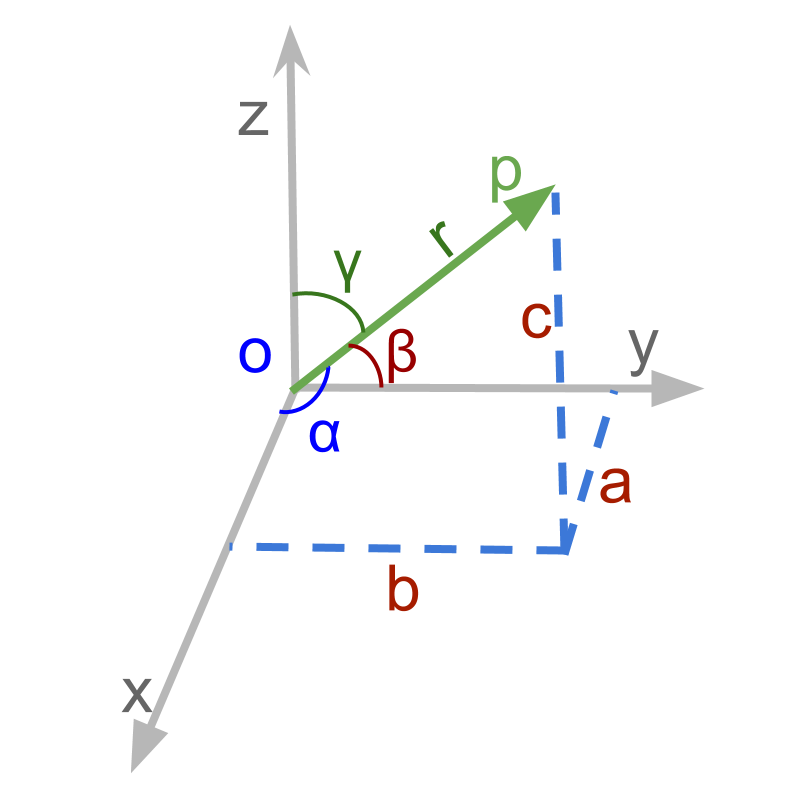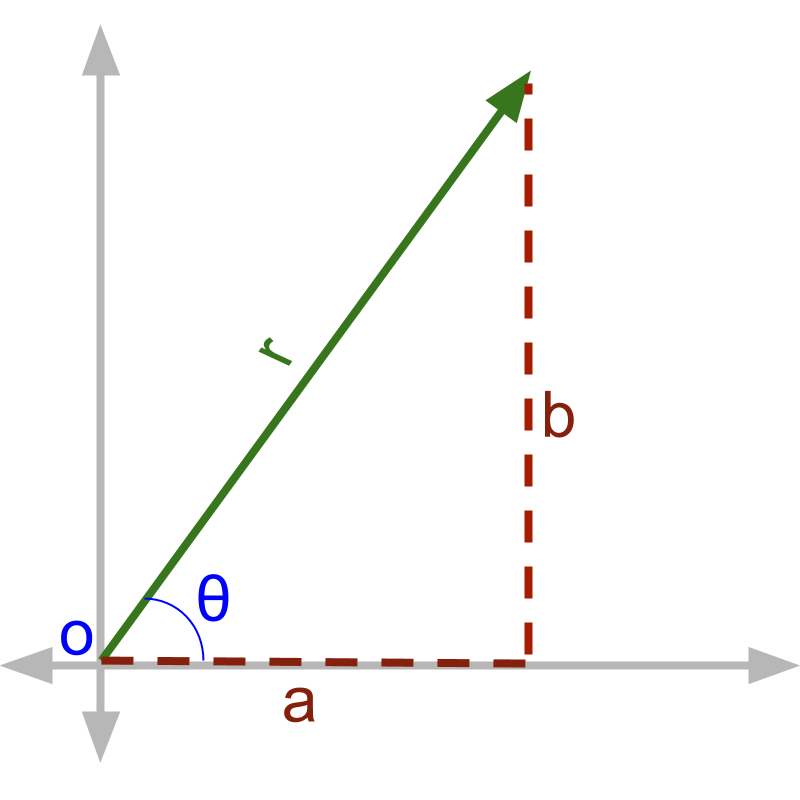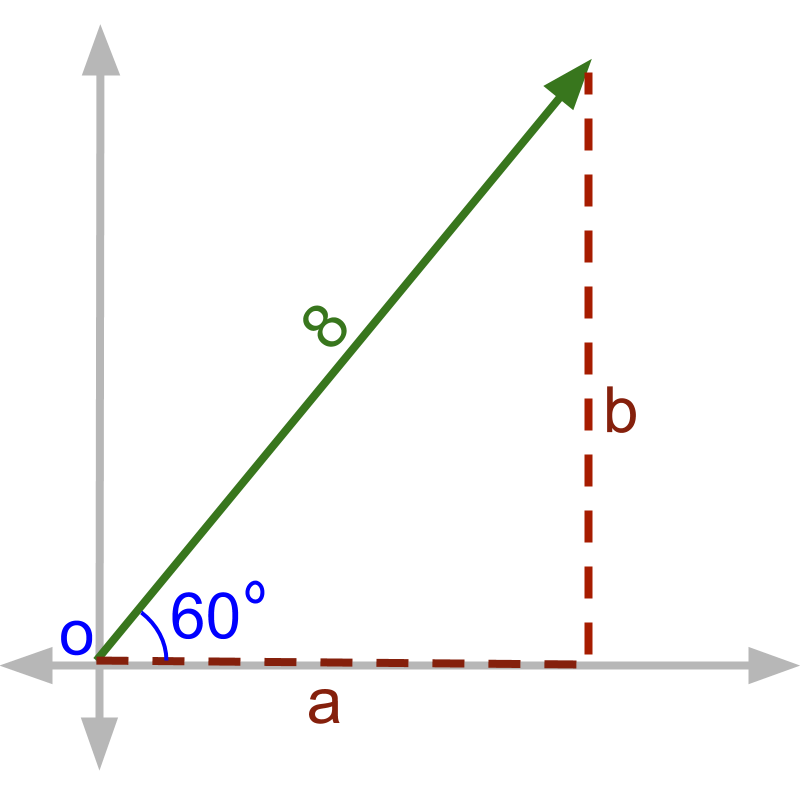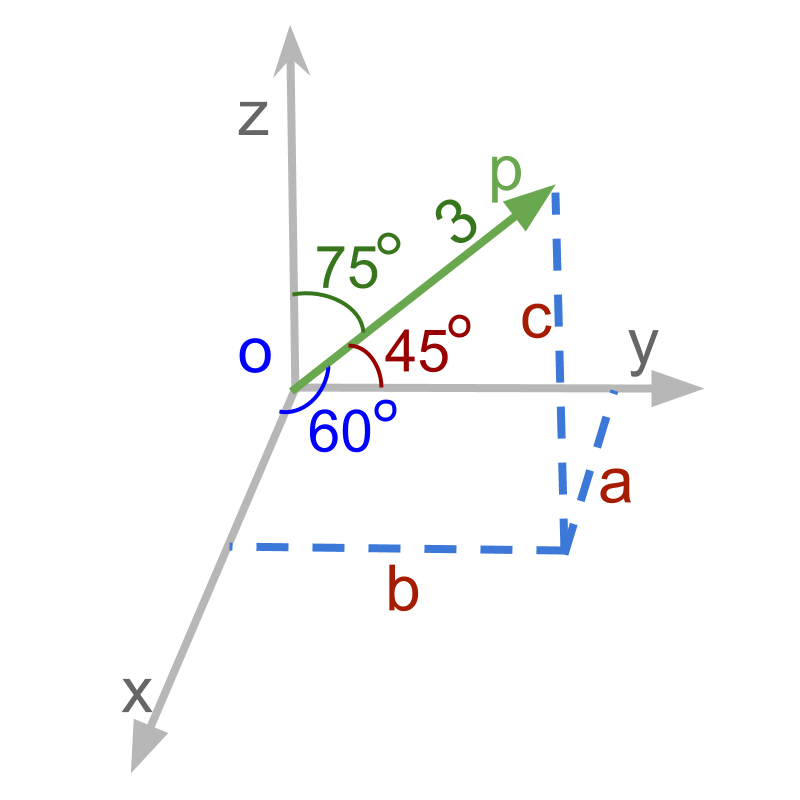maths > vector-algebra

Directional Cosine

what you'll learn...

Overview

Directional Cosine»  Directional cosines:

→  $\stackrel{\to }{p}=ai+bj+ck$$\vec{p} = a i + b j + c k$ makes angles $\alpha ,\beta ,\gamma$$\alpha , \beta , \gamma$ with $x,y,z$$x , y , z$-axes respectively

→  $\mathrm{cos}\alpha =\frac{a}{|p|}$$\cos \alpha = \frac{a}{| p |}$

→  $\mathrm{cos}\beta =\frac{b}{|p|}$$\cos \beta = \frac{b}{| p |}$

→  $\mathrm{cos}\gamma =\frac{c}{|p|}$$\cos \gamma = \frac{c}{| p |}$

alternate representation

'cosine ' of an angle is 'the ratio of adjacent side (adjacent to the given angle) to the hypotenuse in a right angled triangle'.

While referring to vector quantities, we use the form that specifies the components along the axes. eg: $ai+bj+ck$$a i + b j + c k$ specifies the component along $x-$$x -$axis is $a$$a$, the component along $y-$$y -$axis is $b$$b$, and the component along $z-$$z -$axis is $c$$c$.

The alternate representation to component form is to specify magnitude and angles made by the vector on the axes. This has the necessary information about the vector.To describe the vector $ai+bj$$a i + b j$ shown in the figure, if '$r$$r$ and $\theta$$\theta$' are available we can derive the vector form $ai+bj$$a i + b j$.It is noted that the values of $a$$a$ and $b$$b$ in the figure are '$a=8\mathrm{cos}60$$a = 8 \cos 60$ and $b=8\mathrm{sin}60$$b = 8 \sin 60$'.The same in 3D, to describe the vector $ai+bj+ck$$a i + b j + c k$ shown in figure, the vector is completely defined by '$r$$r$ and $\alpha ,\beta$$\alpha , \beta$'. If these three parameters are available we can derive the vector notation $ai+bj+ck$$a i + b j + c k$.

Note that the third angle can be derived from the other two angles.Given the vector in figure, values of $a$$a$, $b$$b$, and $c$$c$ are derived as $a=3\mathrm{cos}60$$a = 3 \cos 60$; $b=3\mathrm{cos}45$$b = 3 \cos 45$; $c=3\mathrm{cos}75$$c = 3 \cos 75$

When a vector with magnitude $r$$r$ and angles $\alpha ,\beta ,\gamma$$\alpha , \beta , \gamma$ is given, the coordinate form of the vector is
$r\left(\mathrm{cos}\alpha i+\mathrm{cos}\beta j+\mathrm{cos}\gamma k\right)$$r \left(\cos \alpha i + \cos \beta j + \cos \gamma k\right)$
$\mathrm{cos}\alpha$$\cos \alpha$, $\mathrm{cos}\beta$$\cos \beta$, $\mathrm{cos}\gamma$$\cos \gamma$ are called the directional cosines of the vector.

Directional cosines: Given that a vector $\stackrel{\to }{p}=ai+bj+ck$$\vec{p} = a i + b j + c k$ makes angles $\alpha ,\beta ,\gamma$$\alpha , \beta , \gamma$ with $x,y,z$$x , y , z$-axes respectively, then the directional cosines of the vector are
$\mathrm{cos}\alpha =\frac{a}{|p|}$$\cos \alpha = \frac{a}{| p |}$
$\mathrm{cos}\beta =\frac{b}{|p|}$$\cos \beta = \frac{b}{| p |}$
$\mathrm{cos}\gamma =\frac{c}{|p|}$$\cos \gamma = \frac{c}{| p |}$

Directional cosines are the ratio of "projections on to an axes" to "the magnitude".

The representation of vectors in the component form is $\stackrel{\to }{p}=ai+bj+ck$$\vec{p} = a i + b j + c k$
Directional cosines along with magnitude provide an alternate representation of a vector.
$\stackrel{\to }{p}=r\left(\mathrm{cos}\alpha i+\mathrm{cos}\beta j+\mathrm{cos}\gamma k\right)$$\vec{p} = r \left(\cos \alpha i + \cos \beta j + \cos \gamma k\right)$

$\mathrm{cos}\alpha =\frac{a}{r}$$\cos \alpha = \frac{a}{r}$

$\mathrm{cos}\beta =\frac{b}{r}$$\cos \beta = \frac{b}{r}$

$\mathrm{cos}\gamma =\frac{c}{r}$$\cos \gamma = \frac{c}{r}$

${r}^{2}={a}^{2}+{b}^{2}+{c}^{2}$${r}^{2} = {a}^{2} + {b}^{2} + {c}^{2}$

${\mathrm{cos}}^{2}\alpha +{\mathrm{cos}}^{2}\beta +{\mathrm{cos}}^{2}\gamma =1$${\cos}^{2} \alpha + {\cos}^{2} \beta + {\cos}^{2} \gamma = 1$

Directional cosines make Unit Vector: For a given vector $ai+bj+ck$$a i + b j + c k$ the directional cosine vector $li+mj+nk$$l i + m j + n k$ is the unit vector in the direction of the given vector. Note that

$l=\mathrm{cos}\alpha =\frac{a}{r}$$l = \cos \alpha = \frac{a}{r}$

$m=\mathrm{cos}\beta =\frac{b}{r}$$m = \cos \beta = \frac{b}{r}$

$n=\mathrm{cos}\gamma =\frac{c}{r}$$n = \cos \gamma = \frac{c}{r}$
This also implies that

${l}^{2}+{m}^{2}+{n}^{2}=1$${l}^{2} + {m}^{2} + {n}^{2} = 1$

examples

What is the magnitude of the directional cosines vector : $\mathrm{cos}\alpha i+\mathrm{cos}\beta j+\mathrm{cos}\gamma k$$\cos \alpha i + \cos \beta j + \cos \gamma k$ ?

The answer is '$1$$1$'. The magnitude = $\sqrt{{\mathrm{cos}}^{2}\alpha +{\mathrm{cos}}^{2}\beta +{\mathrm{cos}}^{2}\gamma }$$\sqrt{{\cos}^{2} \alpha + {\cos}^{2} \beta + {\cos}^{2} \gamma}$, which evaluates to 1.

Find the direction cosines of a line which makes equal angles with the coordinate axes.

$\left(\frac{1}{\sqrt{3}},\frac{1}{\sqrt{3}},\frac{1}{\sqrt{3}}\right)$$\left(\frac{1}{\sqrt{3}} , \frac{1}{\sqrt{3}} , \frac{1}{\sqrt{3}}\right)$ OR $\left(-\frac{1}{\sqrt{3}},-\frac{1}{\sqrt{3}},-\frac{1}{\sqrt{3}}\right)$$\left(- \frac{1}{\sqrt{3}} , - \frac{1}{\sqrt{3}} , - \frac{1}{\sqrt{3}}\right)$

From the question, we know that $\alpha =\beta =\gamma$$\alpha = \beta = \gamma$ and We know the property of directional cosines ${\mathrm{cos}}^{2}\alpha +{\mathrm{cos}}^{2}\beta +{\mathrm{cos}}^{2}\gamma =1$${\cos}^{2} \alpha + {\cos}^{2} \beta + {\cos}^{2} \gamma = 1$

If a line makes angles $45,135,90$$45 , 135 , 90$ with the x, y and z-axes respectively, find its direction cosines.

The answer is '$\mathrm{cos}45,\mathrm{cos}135,\mathrm{cos}90$$\cos 45 , \cos 135 , \cos 90$'.

Find the directional cosine of the vector $2i-3j+\sqrt{3}k$$2 i - 3 j + \sqrt{3} k$.

magnitude of the vector = 4
So directional cosines are $\frac{2}{4},-\frac{3}{4},\frac{\sqrt{3}}{4}$$\frac{2}{4} , - \frac{3}{4} , \frac{\sqrt{3}}{4}$

summary

Directional cosines: Given that a vector $\stackrel{\to }{p}=ai+bj+ck$$\vec{p} = a i + b j + c k$ makes angles $\alpha ,\beta ,\gamma$$\alpha , \beta , \gamma$ with $x,y,z$$x , y , z$-axes respectively, then the directional cosines of the vector are
$\mathrm{cos}\alpha =\frac{a}{|p|}$$\cos \alpha = \frac{a}{| p |}$
$\mathrm{cos}\beta =\frac{b}{|p|}$$\cos \beta = \frac{b}{| p |}$
$\mathrm{cos}\gamma =\frac{c}{|p|}$$\cos \gamma = \frac{c}{| p |}$

Directional cosines are the ratio of "projections on to an axes" to "the magnitude".

Directional cosines make Unit Vector: For a given vector $ai+bj+ck$$a i + b j + c k$ the directional cosine vector $li+mj+nk$$l i + m j + n k$ is the unit vector in the direction of the given vector. Note that

$l=\mathrm{cos}\alpha =\frac{a}{r}$$l = \cos \alpha = \frac{a}{r}$

$m=\mathrm{cos}\beta =\frac{b}{r}$$m = \cos \beta = \frac{b}{r}$

$n=\mathrm{cos}\gamma =\frac{c}{r}$$n = \cos \gamma = \frac{c}{r}$
This also implies that

${l}^{2}+{m}^{2}+{n}^{2}=1$${l}^{2} + {m}^{2} + {n}^{2} = 1$

Outline